# Markov chain

The concept of a Markov chain was developed by Andrey Andreyevich Markov. A Markov chain is a sequence of random variables with the property that it is forgetful of all but its immediate past. For a process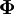${\mathbf \Phi}$ evolving on a space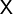${\mathsf X}$ and governed by an overall probability law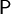${\mathsf P}$ to be a time-homogeneous Markov chain there must be a set of "transition probabilities"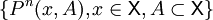$\{P^n (x,A), x \in {\mathsf X}, A \subset {\mathsf X}\}$ for appropriate sets$A$ such that for times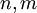$n,m$ in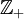${\mathbb Z}_+$ (Ref. 1 Eq. 1.1)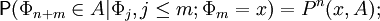${\mathsf P} (\Phi_{n+m} \in A \vert \Phi_j,j \leq m; \Phi_m =x)= P^n(x,A);$
that is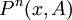$P^n(x,A)$ denotes the probability that a chain at x will be in the set A after n steps, or transitions. The independence of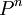$P^n$ on the values of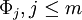$\Phi_j,j \leq m$ is the Markov property, and the independence of$P^n$ and m is the time-homogeneity property.